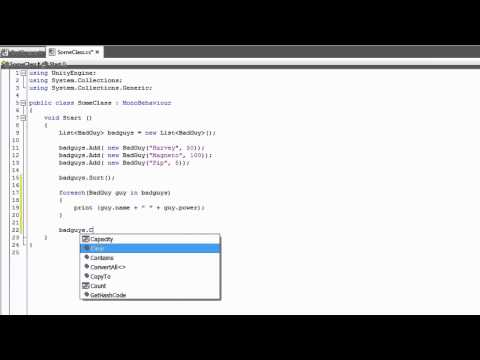# Lists and Dictionaries

#### -

How to create and use the List and Dictionary collections.## Lists and Dictionaries

Intermediário Scripting

### Code snippet

``````using UnityEngine;
using System.Collections;
using System; //This allows the IComparable Interface

//This is the class you will be storing
//in the different collections. In order to use
//a collection's Sort() method, this class needs to
//implement the IComparable interface.
{
public string name;
public int power;

{
name = newName;
power = newPower;
}

//This method is required by the IComparable
//interface.
{
if(other == null)
{
return 1;
}

//Return the difference in power.
return power - other.power;
}
}``````
``````#pragma strict

import System;        // This allows the IComparable Interface.

// This is the class you will be storing
// in the different collections.  In order to use
// a collection's Sort() method, this class needs
// to implement the IComparable interface.
{
public var name : String;
public var power : int;

public function BadGuy (newName : String, newPower : int)
{
name = newName;
power = newPower;
}

// This method is required by the IComparable interface.
public function CompareTo (other : BadGuy)
{
if (other == null)
{
return 1;
}

// Return the difference in power.
return power - other.power;
}
}``````
``````import UnityEngine
import System.Collections
import System

//This allows the IComparable Interface
//This is the class you will be storing
//in the different collections. In order to use
//a collection's Sort() method, this class needs to
//implement the IComparable interface.

public name as string

public power as int

public def constructor(newName as string, newPower as int):
name = newName
power = newPower

//This method is required by the IComparable
//interface.
public def CompareTo(other as BadGuy) as int:
if other is null:
return 1

//Return the difference in power.
return (power - other.power)``````

### Code snippet

``````using UnityEngine;
using System.Collections;
using System.Collections.Generic;

public class SomeClass : MonoBehaviour
{
void Start ()
{
//This is how you create a list. Notice how the type
//is specified in the angle brackets (< >).

{
print (guy.name + " " + guy.power);
}

//This clears out the list so that it is
//empty.
}
}``````
``````#pragma strict

import System.Collections.Generic;

public class SomeClass extends MonoBehaviour
{
function Start ()
{
// This is how you create a list.  Notice how the
// type is specified in the angle brackets (< >).

{
print (guy.name + " " + guy.power);
}

// This clears out the list so that it is empty.
}
}``````
``````import UnityEngine
import System.Collections
import System.Collections.Generic

public class SomeClass(MonoBehaviour):

private def Start():
//This is how you create a list. Notice how the type
//is specified in the angle brackets (< >).

print(((guy.name + ' ') + guy.power))

//This clears out the list so that it is
//empty.

### Code snippet

``````using UnityEngine;
using System.Collections;
using System.Collections.Generic;

public class SomeOtherClass : MonoBehaviour
{
void Start ()
{
//This is how you create a Dictionary. Notice how this takes
//two generic terms. In this case you are using a string and a

//You can place variables into the Dictionary with the

//This is a safer, but slow, method of accessing
//values in a dictionary.
{
//success!
}
else
{
//failure!
}
}
}``````
``````#pragma strict

import System.Collections.Generic;

public class SomeOtherClass extends MonoBehaviour
{
function Start ()
{
// This is how you create a Dictionary. Notice how this takes
// two generic terms.  In this case you are using a string and

var bg1 = new BadGuy("Harvey", 50);
var bg2 = new BadGuy("Magneto", 100);

// You can place variables into the Dictionary with the Add method.

var temp = null;

// This is a safer, but slow, method of accessing values in a dictionary.
// Unityscript dictionaries do not support out parameters like C#, so
// TryGetValue is not available, this is equivalent.
{
}
else
{
// Failure!
}
}
}``````
``````import UnityEngine
import System.Collections
import System.Collections.Generic

public class SomeOtherClass(MonoBehaviour):

private def Start():
//This is how you create a Dictionary. Notice how this takes
//two generic terms. In this case you are using a string and a

//You can place variables into the Dictionary with the

//This is a safer, but slow, method of accessing
//values in a dictionary.